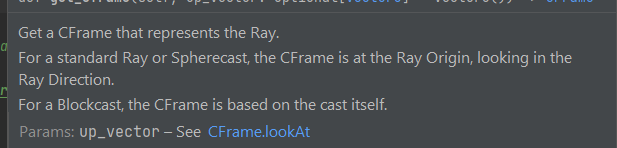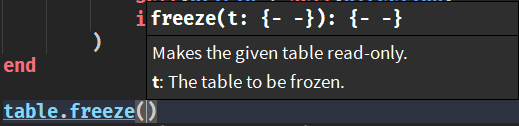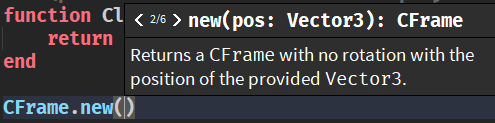# Implement comment-based type, function, method, and variable documentation (i.e. LuauDoc)

Allow programmers to use a system similar to or better to the LuaDoc and SphinxDoc (Python) system.

This would allow programmers to work more easily and more efficiently in large code-bases, as they would be able to peek definitions and documentation of user-made functions, types, and variables in the same way that they do for Roblox types, functions, methods, and objects.

Example of what scripts using “LuauDoc” could look like:

``````--	Documentation example with an online reference / link and a built-in cross-reference.
--	Expected result is the documentation for the CFrame will show on top when hovered over

---	Get a CFrame that represents the Ray.
--	For a standard Ray or Spherecast, the CFrame is at the Ray Origin, looking in the Ray Direction.
--	For a Blockcast, the CFrame is based on the cast itself.
--	@param upVector See [CFrame.lookAt](CFrame.lookAt).
function Class.GetCFrame(self: RayData, upVector: Vector3?): CFrame
return
if self.CastType.Type == "Block" then self.CastType.CFrame
else CFrame.lookAt(
self.Origin,
self.Origin + self.Direction,
if upVector then upVector else Vector3.yAxis
)
end

--	Documentation example with parameter explanation and a cross-reference.
--	Expected result when *clicking* the link would be to move the definition.

---	Project the Ray's CFrame along its direction by a given {distance}.
--	See [GetCFrame](Class.GetCFrame).
--	@param distance The distance to project.
function Class.ProjectCFrame(self: RayData, distance: number): CFrame
return self:GetCFrame() + self:ProjectOffset(distance)
end
``````

Here is a mockup (done in a different editor):Fig. 1

And here are some examples of functions already documented in a similar fashion by roblox:Fig. 2

Fig. 3

Note: this one could be made possible with another Associated Post (or This One)Fig. 4

14 Likes

im surprised this still isnt a feature up to this date. i dont want to switch to Rojo or roblox-ts over the documentation features. i am a dev for some of the games and i utilize OOP a lot. not being able to add documentation where youre writing a method is annoying to say the least. i must check the module documentation either inside the module or inside the website.

big support

2 Likes

This is something we want to add at some point, but I can’t promise when it will come.

5 Likes

Attaching docs to types would be important to carry over for overloaded functions too, i.e.

``````--- Create an identity Quaternion <0, 0, 0, 1>
type QuatIdentity = ()->Quaternion

--- Create a Quaternion from euler angles
-- @param order Order of application of rotations
type QuatEuler = (rot: Vector3, order: Enum.RotationOrder?)->Quaternion

--- Create a Quaternion as a rotation about a global axis
-- @param axis Axis of rotation
-- @param angle Angle of rotation about axis
type QuatAxisAngle = (axis: Vector3, angle: number)->Quaternion

--- Create a Quaternion as a rotation facing toward {lookAt} from {pos}
-- @param pos Source position
-- @param lookAt Target position
-- @param upVec Optional UpVector
type QuatLookAt = (pos: Vector3, lookAt: Vector3, upVec: Vector3?)->Quaternion

--- Create a Quaternion from explicit <x,y,z,w> components
type QuatXYZW = (x: number, y: number, z: number, w: number)->Quaternion

--- Create a Quaternion representation of a CFrame
type QuatCFrame = (cframe: CFrame)->Quaternion

Quaternion.new = (
function(...: any): Quaternion
--....
end
)::(QuatIdentity & QuatEuler & QuatAxisAngle & QuatLookAt  & QuatXYZW & QuatCFrame)

--- Calculate the conjugate of a Quaternion
function Quaternion.Conjugate(self: Quaternion): Quaternion
return Quaternion.new(-self.X, -self.Y, -self.Z, self.W)
end

--- Calculate the inverse of a Quaternion
function Quaternion.Inverse(self: Quaternion): Quaternion
local len: number = self:MagnitudeSqr()
return
if len == 0 then self
else Quaternion.new(-self.X / len, -self.Y / len, -self.Z / len, self.W / len)
end

--- Length of a Quaternion
function Quaternion.Magnitude(self: Quaternion): Quaternion
return math.sqrt(self.X^2 + self.Y^2 + self.Z^2 + self.w^2)
end

--- Length of a Quaternion squared (faster)
function Quaternion.MagnitudeSqr(self: Quaternion): Quaternion
return self.X^2 + self.Y^2 + self.Z^2 + self.w^2
end

--- Returns a normalized Quaternion
function Quaternion.Unit(self: Quaternion): Quaternion
local len: number = self:Magnitude()
return
if len == 1 or len == 0 then self
else Quaternion.new(self.X / len, self.Y / len, self.Z / len, self.W / len)
end

--- Identity Quaternion <0, 0, 0, 1>
Quaternion.identity = Quaternion.new()

type QuatInternal = {X: number, Y: number, Z: number, W: number}

--- [Quaternion](https://en.wikipedia.org/wiki/Quaternion)
-- 4D representation of arbitrary rotations
-- See [Quaternion.new()](Quaternion.new)
export type Quaternion = typeof(
setmetatable(
{} :: QuatInternal,
Metatable
)
)
``````